# NCERT Solutions for Class 7 Maths Chapter 7 Congruence of Triangles

NCERT solutions for class 7 maths chapter 7 Congruence of Triangles- Congruence of triangles is one of the important topics of the geometry. In this chapter, there are two exercises and topic wise practice questions. The solutions of NCERT class 7 chapter 7 congruence of triangles give detailed explanations to all these questions. Students can do their homework easily if they have a tool like CBSE NCERT solutions for class 7 maths chapter 7 congruence of triangles in hand. In geometry, two objects or two figures are congruent if they have the same dimension and same shape, or in other words, we can say that two objects or figures are congruent if both are exact copies of one another. The relation of two objects or two figures being congruent is called congruence. NCERT class 7 maths chapter 7 congruence of triangles deal with plane figures or 2D only, although congruence is a general concept applicable to 3D figures also. Congruence of plane figures, congruence among line segments, congruence of angles, congruence of triangles, some criteria for congruence of triangles like SSS congruence of two triangles, SAS congruence of two triangles, ASA congruence of two triangles, RHS congruence of two right-angled triangles are the concepts which are covered in this chapter. Questions on all these concepts are discussed in the NCERT solutions for class 7 maths chapter 7 congruence of triangles. The NCERT solutions are prepared in such a manner that students are able to understand the concepts easily and prepare themselves very well for CBSE final exams to score higher marks. Here you will get solutions to two exercises of this chapter.

Exercise:7.1

Exercise:7.2

## Topics of NCERT Grade 7 Maths Chapter 7 Congruence of Triangles-

7.1 Introduction

7.2 Congruence of Plane Figures

7.3 Congruence among Line Segments

7.4 Congruence of Angles

7.5 Congruence of Triangles

7.6 Criteria for Congruence of Triangles

7.7 Congruence among Right-Angled Triangles

## 1.   When two triangles, say ABC and PQR are given, there are, in all, six possible matchings or correspondences. Two of them are:

and

Find the other four correspondences by using two cutouts of triangles. Will all these correspondences lead to congruence? Think about it.

the other four correspondences by using two cutouts of triangles are :

## NCERT solutions for class 7 chapter 7 congruence of triangles topic 7.6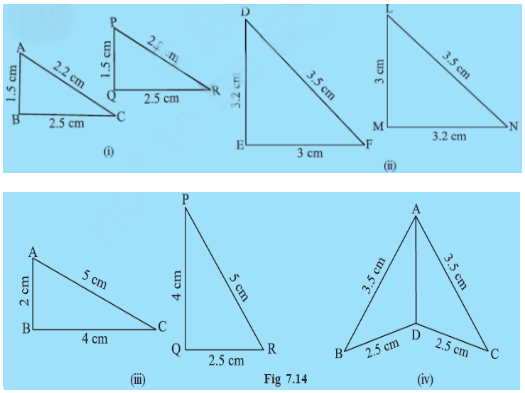i) Since

AB = PQ

BC = QR

CA = PR

So, by SSS congruency rule both triangles are congruent to each other.

ii) Since,

ED = MN

DF = NL

FE = LM

So, by SSS congruency rule both triangles are congruent to each other.

.

iii) Since

AC = PR

BC = QR But

So the given triangles are not congruent.

iv) Since,

AB = AC

BD = CD

So, By SSS Congruency rule, they both are congruent to each other.

.

(i) State the three pairs of equal parts in  and
(ii) Is  Give reasons.
(iii) Is  Why?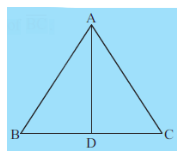Here in   and

i) Three pair of equal parts are:

BD = CD ( as d is the mid point of BC)

AB = AC (given in the question)

ii) Now,

by SSS Congruency rule,

iii) As both triangles are congruent to each other we can compare them and say

.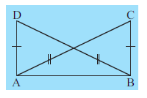Given,

and

.

AB = AB ( common side )

So By SSS congruency rule,

.

So this statement is meaningfully written as all given criterions are satisfied in this.

## 1.  ABC is an isosceles triangle with  (Fig 7.17). Take a trace-copy of  and also name it as  (i) State the three pairs of equal parts in . (ii) Is ? Why or why not? (iii) Is  ? Why or why not?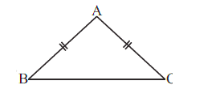Here, in .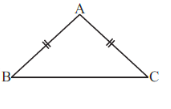i)the three pairs of equal parts in  are

AB = AC

BC = CB

AC = AB

ii)

Hence By SSS Congruency rule, they both are congruent.

iii) Yes,  because  are congruent and by equating the corresponding parts of the triangles we get,

.

Since both the sides    and intersects at E,

is included between the sides   and of  .

To prove congruency by SAS rule, we need to equate two corresponding sides and one corresponding angle,

so in   proving  we need,

And

.

Hence the extra information we need is .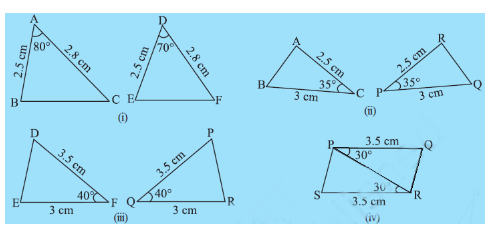i) in  and

AB = DE

AC = DF

Hence, they are not congruent.

ii) In  and

AC = RP = 2.5 cm

CB = PQ = 3 cm

Hence by SAS congruency rule, they are congruent.

.

iii) In  and

DF= PQ = 3.5 cm

FE= QR = 3 cm

Hence, by SAS congruency rule, they are congruent.

iv) In  and

QP = SR = 3.5 cm

PR = RP (Common side)

Hence, by SAS congruency rule, they are congruent.

.

The side MN is the side which is included between the angles and N of .

## 2. You want to establish , using the ASA congruence rule. You are given that and. What information is needed to establish the congruence? (Draw a rough figure and then try!)

As we know, in ASA congruency two angles and one side is equated to their corresponding parts. So

To Prove

And The side joining these angles is

.

So the information that is needed in order to prove congruency is .

(i) State the three pairs of equal parts in two triangles and .

(ii) Which of the following statements are true?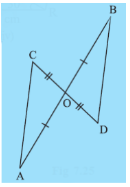i) The three pairs of equal parts in two triangles and  are:

CO = DO (given)

OA = OB (given )

( As opposite angles are equal when two lines intersect.)

ii) So by SAS congruency rule,

that is

Hence, option B is correct.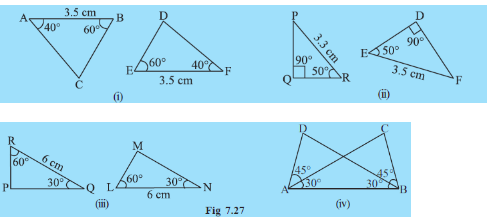i) in  and

AB = FE = 3.5 cm

So by ASA congruency rule, both triangles are congruent.i.e.

ii) in  and

But,

So, given triangles are not congruent.

iii) in  and

RQ = LN = 6 cm

So by ASA congruency rule, both triangles are congruent.i.e.

.

iv) in  and

AB = BA (common side)

So by ASA congruency rule, both triangles are congruent.i.e.

## 4. Given below are measurements of some parts of two triangles. Examine whether the two triangles are congruent or not, by ASA congruence rule. In the case of congruence, write it in symbolic form.

i)

Given in  and  .

So, by ASA congruency criterion, they are congruent to each other.i.e.

.

ii)

Given in  and  .

For congruency by ASA criterion, we need to be sure of equity of the side which is joining the two angles which are equal to their corresponding parts. Here the side QR is not given which is why we cannot conclude the congruency of both the triangles.

iii)

Given in  and  .

For congruency by ASA criterion, we need to be sure of equity of the side which is joining the two angles which are equal to their corresponding parts. Here the side QR is not given which is why we cannot conclude the congruency of both the triangles.

(i) State the three pairs of equal parts in triangles  and .
(ii) Is  Give reasons.
(iv) Is  Give reasons.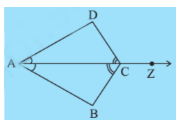i)

Given in triangles  and

( common side)

ii)

So, By ASA congruency criterion,triangles  and  are congruent.

iii)

Since  , all corresponding parts will be equal. So

.

iv)

Since  , all corresponding parts will be equal. So

## 1.  In Fig 7.32, measures of some parts of triangles are given.By applying RHS congruence rule, state which pairs of triangles are congruent. In case of congruent triangles, write the result in symbolic form.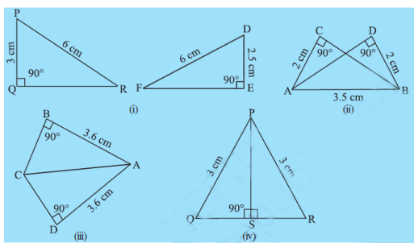i) In  and

Hence they are not congruent.

ii)

In  and

( same side )

So, by RHS congruency rule,

iii)

In  and

( same side )

So, by RHS congruency rule,

iv)

In  and

( same side )

So, by RHS congruency rule,

and

To prove congruency by RHS (Right angle, Hypotenuse, Side ) rule, we need hypotenuse and side equal to the corresponding hypotenuse and side of different angle.

So Given

( Right angle )

( Side )

So the third information we need is the equality of  Hypotenuse of both triangles. i.e.

Hence, if this information is given then we can say,

.

(i) State the three pairs of equal parts in and .
(ii) Is  ? Why or why not?
(iii) Is  ? Why or why not?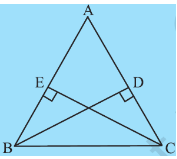i) Given,  in and .

ii) So, By RHS Rule of congruency, we conclude:

iii) Since both the triangle are congruent, all parts of one triangle are equal to their corresponding part from another triangle.

So.

.

(i) State the three pairs of equal parts in and .
(ii) Is ? Why or why not?
(iii) Is ? Why or why not?
(iv) Is ? Why or why not?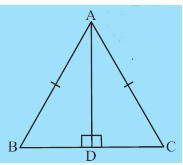i) Given in and .

( Common side)

ii) So, by RHS Rule of congruency, we conclude

iii) Since both triangles are congruent all the corresponding parts will be equal.

So,

iv) Since both triangles are congruent all the corresponding parts will be equal.

So,

.

## NCERT solutions for class 7 maths chapter 7 congruence of triangles exercise 7.1

(a) Two line segments are congruent if ___________.
(b) Among two congruent angles, one has a measure of ; the measure of the other angle is ___________.
(c) When we write , we actually mean ___________.

a)Two line segments are congruent if they are identical in shape and size and which is the case when the length of two line segments are equal.

b)  As the congruent things are a photocopy of each other.

c) When we write , We mean that both the angles(A & B) are equal.

Any two things that have identical shape and size are congruent like all the same kind of pens are congruent to one another. every same kind of bench in class are congruent to one another.all the similar football is congruent to one another.

Corresponding parts of the two congruent triangles are :

Sides:

Angles:

Given,

The part of  that correspond to

## CBSE NCERT solutions for class 7 maths chapter 7 congruence of triangles exercise 7.2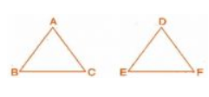Since we are comparing all the sides of two triangles, The SSS (side, side, side) Congruent criterion is used.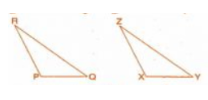Since we are comparing two sides and one angle of the two triangles, the SAS (sie, angle, side) congruent criterion is used to prove them congruent.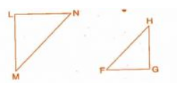Since we are comparing two angles and one side, ASA(Angle, Side, Angle) congruency criterion is used to prove the congruency.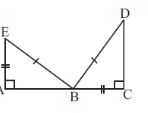Since we are comparing two sides and one angle of the two triangles, the SSA (Side, Side, Angle) congruent criterion is used to prove the congruency.

(a) If you have to use  criterion, then you need to show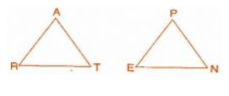As we know that in the criterion of proving congruent, all three corresponding sides are equal to another. So to prove the congruency, we kneed to know the following things:

(b) If it is given that  and you are to use SAS criterion, you need to have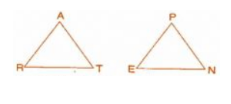As we know in SAS  criterion the two sides and one angle are identical to their corresponding parts of another triangle. So to prove congruency we need to prove that,

(c) If it is given that  and you are to use ASA criterion, you need to have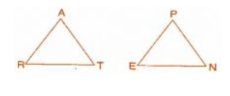Given,

also,

Now, As we know in the ASA criterion of proving congruency, the one and side two angles are equal to their corresponding parts. So,

 Steps Reasons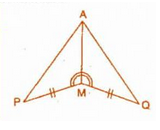Steps Reasons Given in the question Given in the question. the side which is common in both triangle By SAS Congruence Rule

In , and . A student says that by AAA congruence criterion. Is he justified? Why or why not?

No, because it is not necessary that two triangles will be congruent if their all three corresponding angles are equal. in this case, the triangles might be zoomed copy of one another.

## 5. In the figure, the two triangles are congruent. The corresponding parts are marked. We can write ?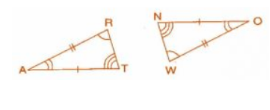Comparing from the figure.

By SAS Congruency criterion, we can say that

]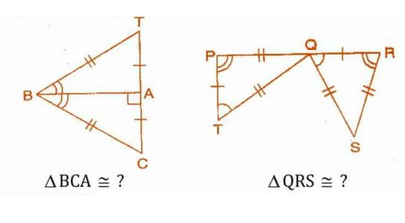Comparing from the figure, we get,

So By SSS Congruency Rule,

Also,

Comparing from the figure, we get,

So By SSS Congruency Rule,

.

What can you say about their perimeters?

When two triangles are congruent, the corresponding parts are exactly identical so they have the same area and perimeter.

While the triangles are not congruent but have the same area, then the perimeter of both triangles are not equal.

Five pairs of congruent parts can be three pairs of sides and two pairs of angles. In that case, the SAS or ASA criterion would prove them to be congruent. Hence, such a figure is not possible.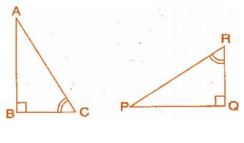Given

One additional pair which is not given in the figure is

We used the ASA Criterion as the two corresponding angles are given and we figured out the side by congruency.

10.  Explain, why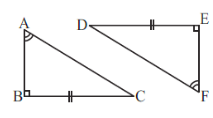Comparing both triangles, we have,

So By RHS congruency criterion,

.

## NCERT Solutions for Class 7 Maths - Chapter-wise

 Chapter No. Chapter Name Chapter 1 Solutions of NCERT for class 7 maths chapter 1 Integers Chapter 2 CBSE NCERT solutions for class 7 maths chapter 2 Fractions and Decimals Chapter 3 NCERT solutions for class 7 maths chapter 3 Data Handling Chapter 4 Solutions of NCERT for class 7 maths chapter 4 Simple Equations Chapter 5 CBSE NCERT solutions for class 7 maths chapter 5 Lines and Angles Chapter 6 NCERT solutions for class 7 maths chapter 6 The Triangle and its Properties Chapter 7 NCERT solutions for class 7 maths chapter 7 Congruence of Triangles Chapter 8 NCERT solutions for class 7 maths chapter 8 comparing quantities Chapter 9 CBSE NCERT solutions for class 7 maths chapter 9 Rational Numbers Chapter 10 NCERT solutions for class 7 maths chapter 10 Practical Geometry Chapter 11 Solutions of NCERT for class 7 maths chapter 11 Perimeter and Area Chapter 12 CBSE NCERT solutions for class 7 maths chapter 12 Algebraic Expressions Chapter 13 NCERT solutions for class 7 maths chapter 13 Exponents and Powers Chapter 14 Solutions of NCERT for class 7 maths chapter 14 Symmetry

## Important points of NCERT Class 7 Maths Chapter 7 Congruence of Triangles-

Questions discussed in the NCERT solutions for class 7 maths chapter 7 congruence of triangles are based on the following congruence criteria.

• SSS Congruence of two triangles- Under a given correspondence, two triangles are congruent if the three sides of the one triangle are equal in measure to the three corresponding sides of the other triangle.
• SAS Congruence of two triangles- Under a given correspondence, two triangles are congruent if two sides and the angle included between them in one of the triangles are equal in measures to the corresponding sides and the angle included between them of the other triangle.
• ASA Congruence of two triangles- Under a given correspondence, two triangles are congruent if two angles and the side included between them in one of the triangles are equal in measures to the corresponding angles and the side included between them of the other triangle.
• RHS Congruence of two right-angled triangles- Under a given correspondence, two right-angled triangles are congruent if the hypotenuse and a leg of one of the triangles are equal to the hypotenuse and the corresponding leg of the other triangle.

Same questions described in the  NCERT solutions for class 7 maths chapter 7 congruence of triangles can be expected for exams.